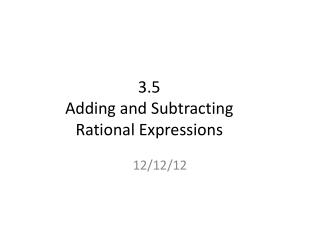DownloadDownload Presentation3.5 Adding and Subtracting Rational Expressions

# 3.5 Adding and Subtracting Rational Expressions

Télécharger la présentation## 3.5 Adding and Subtracting Rational Expressions

- - - - - - - - - - - - - - - - - - - - - - - - - - - E N D - - - - - - - - - - - - - - - - - - - - - - - - - - -
##### Presentation Transcript

1. 3 5 x • • Divide out the common factor 5 and simplify. 3x = = 5 x 5 + b. x 6x 2 3 x 3 • • c. Divide out the common factors 2 and x. = = 14x2 2 7 x x 7x • • • Example 1 Simplify a Rational Expression Simplify the rational expression, if possible. 15x a. 5 This expression is already in simplest form.

2. Ex 1: Simplify = Factor out 3xy

3. Ex 2: Simplify = Factor out (x+5) = Combine Like Terms

4. Ex 3: Simplify Big X = Factor out () Simplify brackets = a2 – b2

5. Different Denominators: When adding/subtracting fractions and the denominators are different, find the least common denominator (LCD).

6. Ex 4: Simplify LCD:

7. Ex 5: Simplify Big X LCD: ( Simplify numerator

8. Ex 6: Simplify Big X LCD: ( Switch the signs of the second numerator Combine Like terms

9. Homework: WS 3.5 odd only